Chapter 10.5, Problem 61EFinite Mathematics and Applied Cal...

7th Edition
Stefan Waner + 1 other
ISBN: 9781337274203

Solutions

Chapter
SectionFinite Mathematics and Applied Cal...

7th Edition
Stefan Waner + 1 other
ISBN: 9781337274203
Textbook Problem

In Exercises 59–64, match the graph of f to the graph of f ′ . (The graphs of f ' are shown after Exercise 64.)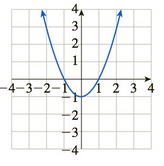Graph of derivatives for Exercises 59-64:(A)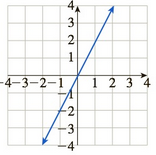(B)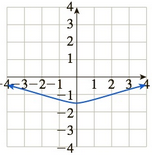(C)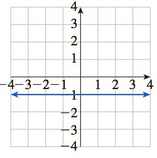(D)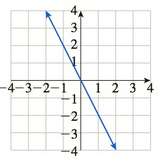(E)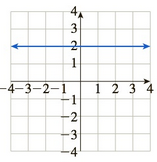(F)To determine

The graph of f of f from the provided graphs.

The graph of f is: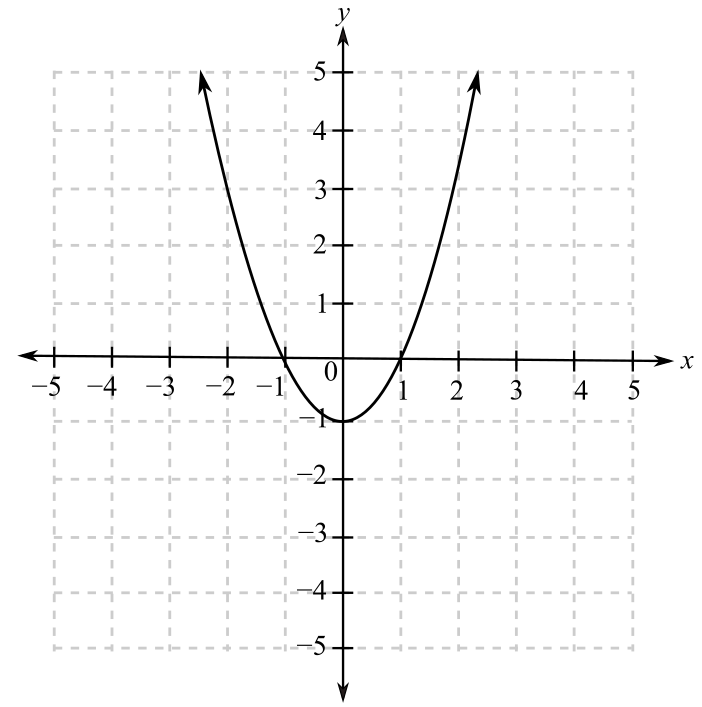The graphs provided for f are: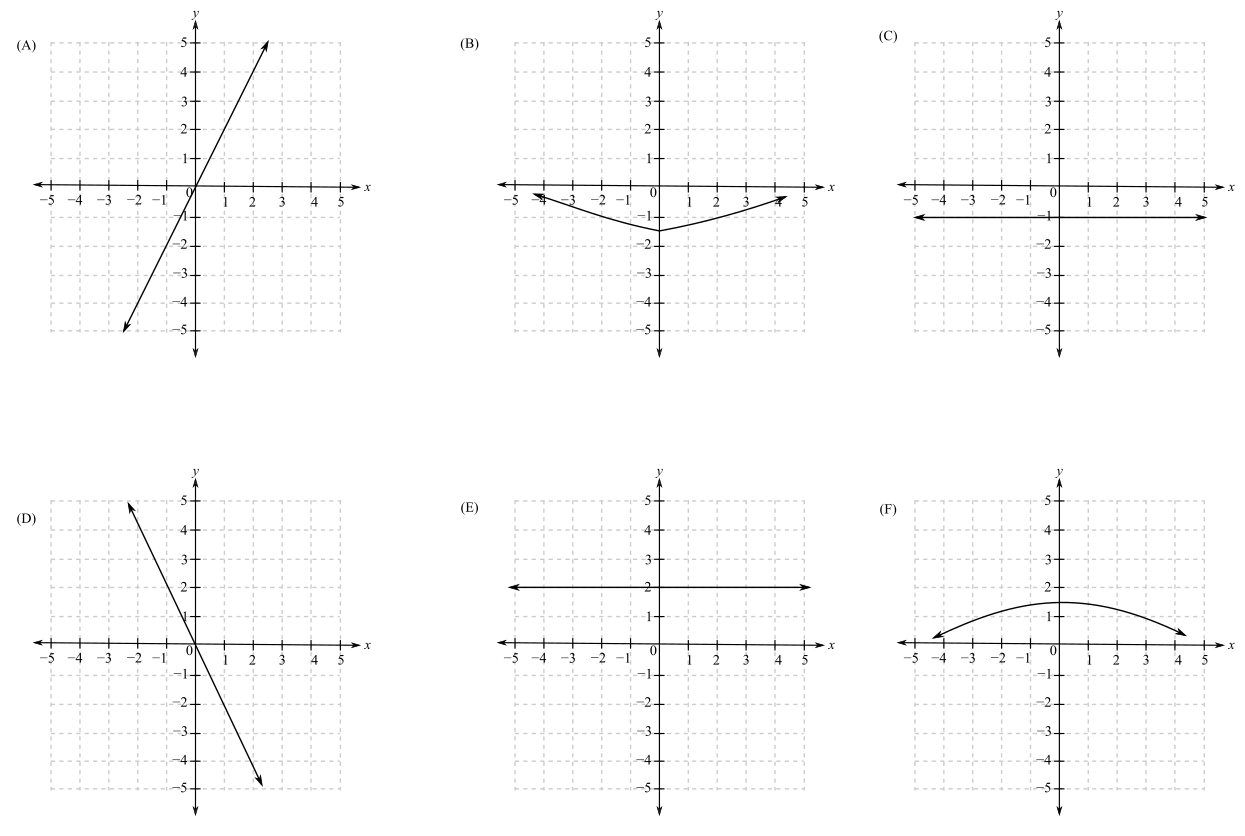Explanation

Given Information:

The graph of f is:

The graphs provided for f are:

When a function is increasing or rising, the slope of the function is positive and greatest where the tangent is more slanted whereas when the function is decreasing or falling, its slope is negative and it has the least value where the tangent is the most slanted

Still sussing out bartleby?

Check out a sample textbook solution.

See a sample solution

The Solution to Your Study Problems

Bartleby provides explanations to thousands of textbook problems written by our experts, many with advanced degrees!

Get Started

In Exercises 31-34, find an equation of the line that passes through the points. 33. (1, 2) and (3, 2)

Applied Calculus for the Managerial, Life, and Social Sciences: A Brief Approach

47. (a) Find the market equilibrium. (b) Find the consumers surplus at market equilibrium.

Mathematical Applications for the Management, Life, and Social Sciences

Evaluate the integral, if it exists. 0T(x48x+7)dx

Single Variable Calculus: Early Transcendentals

Simplified, log3 9x3 is: 2 + 3 log3 x 6 log3 x 9 log3 x 9 + log3 x

Study Guide for Stewart's Single Variable Calculus: Early Transcendentals, 8th

converges to: 2 the series diverges

Study Guide for Stewart's Multivariable Calculus, 8th